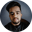Related Tags

kotlin
commmunitycreator

# What are the jump expressions in Kotlin?Shubham Singh Kshatriya

## Overview

The jump expressions in Kotlin are used to control the flow of the execution of the program. Kotlin supports the following jump expressions:

• return
• break
• continue

### 1. The return expression

The return expression returns some value generated through an expression or value of a variable or a constant value.

This expression is often used to return values from a function after some execution.

### Example

In the following code snippet, we can see the use of the return expression in Kotlin:

fun sum(n1: Int, n2: Int): Int {
var result: Int = n1 + n2

// use of return expression
return result
}

fun main(args : Array<String>) {
var result = sum(5, 10)
println(result)
}

Example of return expression

### Explanation

In the above code snippet:

• Lines 1 to 6: We define a function sum() that accepts two parameters, n1 and n2, calculates its sum and returns the result using the return expression in line 5.
• Lines 8 to 11: We invoke the function sum()inside the function main(), and print the result to the console.

### 2. The break expression

The break expression is used to terminate the closest enclosing loop.

If there are multiple nested loops, and the break expression is in the most inner-loop, this expression will terminate only the most inner-most loop .

### Example

In the following code snippet, we can see the use of the break expression in Kotlin:

fun main(args : Array<String>) {
var n: Int = 6
for (i: Int in 1..n) {
// use of break expression
if (i == 4)
break

println("The value of i is: $i") } } Example of break expression ### Explanation In the above code snippet, inside the main() function: • Line 2: We declare a variable n and assign it some value. • Lines 4 to 12: We use a nested to loop and print the value of i. • Line 7: We use the break expression to terminate the inner-loop if i = 4. ### 3. The continue expression The continue expression is very similar to the break expression. The only difference is that the continued expression will only break or skip the current iteration. ### Example In the following code snippet, we can see the use of the continue expression in Kotlin: fun main(args : Array<String>) { var n: Int = 6 for (i: Int in 1..n) { // use of break expression if (i == 4) continue println("The value of i is:$i")
}
}
Example of continue expression

### Explanation

In the above code snippet, inside the main() function:

• Line 2: We declare a variable n and assign it some value.
• Lines 4 to 12: We use a nested to loop and print the value of i.
• Line 7: We use the continue expression to skip the iteration if i = 4.

RELATED TAGS

kotlin
commmunitycreator

CONTRIBUTORShubham Singh Kshatriya
RELATED COURSES

View all Courses

Keep Exploring

Learn in-demand tech skills in half the time